# 如何计算滚珠丝杠的电机驱动扭矩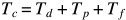Tc = 恒速扭矩 (Nm)

Td = 驱动负载的扭矩 (Nm)

Tp = 预紧扭矩（制造商提供）（Nm

Tf = 支撑轴承和密封件摩擦产生的扭矩（由制造商提供）（Nm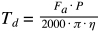Fa = 总轴向力 (N)

P = (mm)

η = 滚珠丝杠效率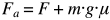F = 轴向过程力 (N)

m = 移动的质量 (kg)

g = 重力加速度 (m/s2)

μ = 直线导轨的摩擦系数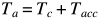Ta = 加速期间的总扭矩 (Nm)

Tacc = 加速度产生的扭矩 (Nm)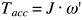J = 系统惯量 (kgm2)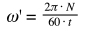N = 角速度 (rpm)

t = 加速时间（s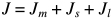Jm = 电机惯量（制造商提供）（kgm2

Js = 丝杆惯量（厂家提供）（kgm2

Jl = 负载惯量 (kgm2)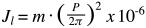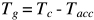×